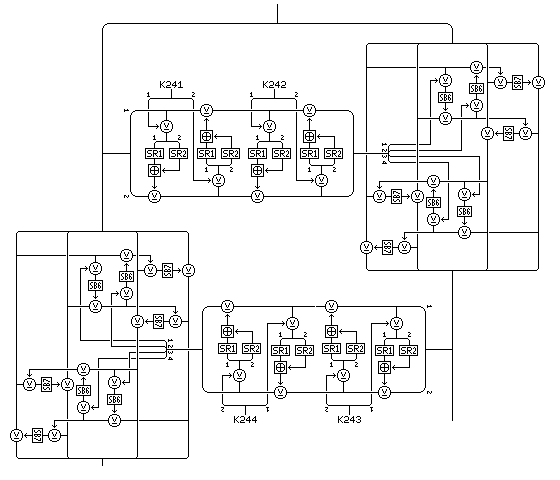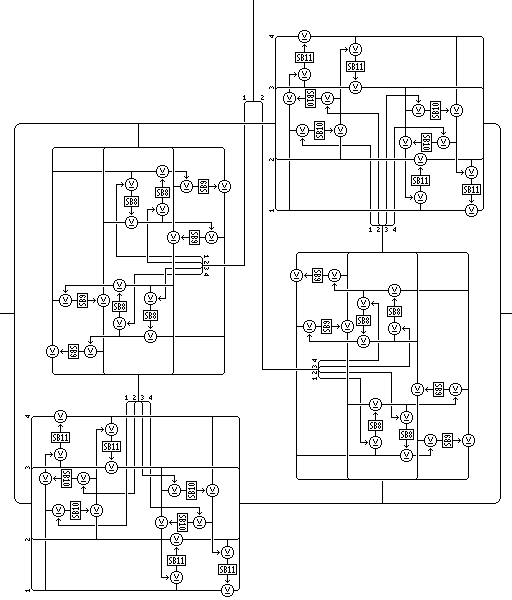[Next] [Up] [Previous] [Index]

# Quadibloc 2002E: The f-function of the Core Rounds

The 128-bit intermediate result output from the Feistel rounds of the Quadibloc II type that are applied to the left half of the block, after undergoing the bit swap operation shown on the previous page, is then modified by Feistel rounds, rather than by Quadibloc 2002A rounds, as were used in Quadibloc 2002D. The f-function for these rounds, as shown below:has a structure of the "Fractal Feistel" type, with only the innermost portion being based on the "GoodStuff" round type introduced in Quadibloc III with S10 and S11 replaced by SR1 and SR2, for consistency. Placing this structure only in the innermost part of the f-function reduces the potential danger from the possibility that these purely random S-boxes SR1 and SR2 might be biased.

Two rounds are shown in the diagram; the f-function uses four rounds, and the round of which that f-function is the f-function occurs four times. Thus, in addition to using subkeys K192 through K197 in the Quadibloc f-functions that act on the left half of the block and produce an intermediate result, in the f-function applied to the intermediate result, the first core round uses 32-bit subkeys K241 through K272.

The output from the full four rounds of this 64-bit f-function is then applied to the other 64-bit half of the 128-bit quantity derived from the intermediate results of the operations on the left half of the original block by the following combiner:Four rounds with the four-round f-function shown above and this combiner constitute the overall f-function for the cryptographic core round.

Once again, the fractal Feistel structure is employed, to produce a high level of indirection.

There are two weaknesses in this cipher design as it stands, despite its extreme complexity, and likely high strength. The innermost part of the f-function, using SR1 and SR2, is vulnerable to bias in these S-boxes; if they are biased, the 32 subkeys for the round will end up having a more limited effect on the f-function. Also, while the input to the f-function is 128 bits long, it is fully derived from a 64-bit half of the block and fixed subkeys, so for any one round of the cipher, it has only 2^64 possible inputs, and 2^64 possible outputs, so for any one fixed key, part of its potential is wasted. This indicates that the cipher could be strengthened, if worked into a stream cipher of some sort, if the subkeys used for the Quadibloc II type rounds, K193 through K240, were varied with each block enciphered.

Two subkeys at a time are applied using the two unconstrained key-dependent S-boxes SR1 and SR2 in an operation that takes a 16-bit input and produces a 16-bit output and proceeds as follows:

A quantity is formed from the XOR of the left half of the first subkey for the operation and the left half of the input. The right half of the input is XORed with the modulo-65536 sum of the entry in SR1 indexed by the left byte of this quantity and the entry in SR2 indexed by the right byte of this quantity.

Then, a quantity is formed from the XOR of the right half of the first subkey for the operation and the right half of the input as currently modified. The left half of the input is XORed with the modulo-65536 sum of the entry in SR1 indexed by the left byte of this quantity and the entry in SR2 indexed by the right byte of this quantity.

Then the input, as now modified, undergoes those two rounds again, but with the left and right halves of the second subkey for the operation taking the place of the left and right halves of the first subkey for the operation.

This operation will be called the inner Feistel cipher.

The rest of this part of the cipher is all built from the combiner introduced in Quadibloc XI, which operates as follows:

Considering the four bytes of the input as I1, I2, I3, and I4, and the four bytes of the modification input, used as subkeys, as M1, M2, M3, and M4, and the two S-boxes used by the cipher as SA and SB, the combiner consists of the following steps:

• T1 = I1
• T2 = I2
• T2 = T2 xor SA( M1 xor T1 )
• T1 = T1 xor SA( M2 xor T2 )
• I4 = I4 xor SB( T1 xor I3 )
• I3 = I3 xor SB( T2 xor I4 )
• T1 = I3
• T2 = I4
• T2 = T2 xor SA( M3 xor T1 )
• T1 = T1 xor SA( M4 xor T2 )
• I2 = I2 xor SB( T1 xor I1 )
• I1 = I1 xor SB( T2 xor I2 )

This shall be known as the combiner module operation.

The cipher Quadibloc 2002D uses groups of four cryptographic core rounds, which we are describing here. The f-function for these rounds will be called the master f-function, and it has a 128-bit input and a 128-bit output. It is composed of four rounds, these will be called layer rounds, having a layer f-function with a 64-bit input and a layer combiner. The layer f-function is itself composed of four rounds, which will be called segment rounds, composed of a segment f-function with a 32-bit input and output, and which uses two 32-bit subkeys; that f-function being the inner Feistel cipher described above, and the segment combiner consists of the combiner module operation described above.

The f-function with a 64-bit input and a 64-bit output that is used for one of four rounds of the f-function of the round as a whole, or the layer f-function, as named above, operates as follows:

• A copy of the left half of the 64-bit input to the layer f-function is used as the input to an inner Feistel cipher using the first two subkeys for the layer round. The 32-bit output from this, the segment f-function, is used as the modification input to a combiner module operation, using key-dependent S-boxes SB6 and SB7 as its two S-boxes, and taking the right half of the copy of the left half of the 64-bit input as its input, and then providing a modified form of that as its output.

This constitutes the first segment round.

The three remaining segment rounds proceed similarly, each one using two succeeding 32-bit subkeys, and each one (operating in place, without swapping halves) exchanging the roles of the left and right halves of the 64-bit input to the layer f-function.

The layer combiner then applies, in the first layer round, the 64-bit output of the layer f-function to a 64-bit value.

First, it takes the first 32 bits of its 64-bit modification input, which is the output of the layer f-function, and uses them as the modification input to a combiner module operation which takes as its input a copy of the left half of the 64-bit value being modified, and which uses S-boxes SB8 and SB9. The output of this combiner module operation is used as the modification input to another combiner module operation, using S-boxes SB10 and SB11, which takes the right half of the 64-bit value as its input, modifying it by replacing it with its output.

Then the layer combiner takes the last 32 bits of the 64-bit modification input to the layer combiner, the output of the layer f-function, and uses them as a modification input to a combiner module operation, using S-boxes SB8 and SB9, which takes as its input a copy of the right half of the 64-bit value being modified, in its current modified state. The output is used as the modification input to another combiner module operation, using S-boxes SB10 and SB11, which takes the left half of the 64-bit value to be modified as its input, and modifies it by supplying its output in its stead.

The master f-function consists of four layer rounds. In the first layer round, the left half of the 128-bit input is used as the input to the layer f-function, and the right half of the 128-bit input is used as the 64-bit value to be modified by the layer combiner. The role of the two halves alternates between layer rounds, but this is done in place (the equivalent of an extra swap halves after the last round).

[Next] [Up] [Previous] [Index]